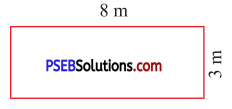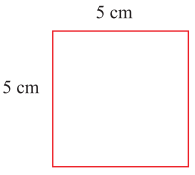# PSEB 5th Class Maths Solutions Chapter 8 Perimeter and Area Ex 8.1

Punjab State Board PSEB 5th Class Maths Book Solutions Chapter 8 Perimeter and Area Ex 8.1 Textbook Exercise Questions and Answers.

## PSEB Solutions for Class 5 Maths Chapter 8 Perimeter and Area Ex 8.1

1. Find the perimeter :

Question 1.Solution:
Perimeter of a rectangle = Length + Breadth + Length + Breadth
= 2 (8 m + 3 m)
= 2 × 11 m
= 22 m.Question 2.Solution:
Perimeter of a square = side + side + side + side
= 4 × side
= 4 × 5 cm
= 20 cm.

2. Find the perimeter of the rectangle whose length and breadth are as follows :

Question 1.
3 cm, 2 cm
Solution:
Length of the rectangle = 3 cm
Breadth of the rectangle = 2 cm
Perimeter of the rectangle = 2 (Length + Breadth)
= 2 (3 cm + 2 cm)
= 2 × 5 cm
= 10 cm.

Question 2.
12 m, 10 m
Solution:
Length of the rectangle = 12 m
Breadth of the rectangle = 10 m
Perimeter of the rectangle = 2 (Length + Breadth)
= 2 (12 m + 10 m)
= 2 × 22 m
= 44 m.Question 3.
15 cm, 8 cm.
Solution:
Length of the rectangle = 15 cm
Breadth of the rectangle = 8 cm
Perimeter of the rectangle = 2 (Length + Breadth)
= 2 (15 cm +8 cm)
= 2 × 23 cm
= 46 cm.

3. Find the perimeter of the square, whose side is :

Question 1.
4 cm
Solution:
Side of the square = 4 cm
Perimeter of the square = 4 × side
= 4 × 4 cm
= 16 cm.

Question 2.
8 cm
Solution:
Side of the square = 8 cm
Perimeter of the square = 4 × side
= 4 × 8 cm
= 32 cm.

Question 3.
10 cm
Solution:
Side of the square = 10 cm
Perimeter of the square = 4 × side
= 4 × 10 cm
= 40 cm.Question 4.
72 mm
Solution:
Side of the square = 72 mm
Perimeter of the square = 4 × side
= 4 × 72 mm
= 288 mm.

4. Find the side of the square whose perimeter is :

Question 1.
48 cm
Solution:
Perimeter of the square = 48 cm
Side of the square = $$\frac{\text { Perimeter }}{4}$$
= $$\frac{48}{4}$$ cm
= 12 cm

Question 2.
80 m
Solution:
Side of the square = $$\frac{\text { Perimeter }}{4}$$
= $$\frac{80}{4}$$ cm
= 20 cm

Question 3.
24 m
Solution:
Side of the square = $$\frac{\text { Perimeter }}{4}$$
= $$\frac{24}{4}$$ cm
= 6 cmQuestion 5.
The length and breadth of a rectangular park is 96 m and 64 m respectively. Find the length of wire which can fence it all around.
Solution:
Length of rectangular park = 96 m
Breadth of rectangular park = 64 m
Perimeter of rectangular park = 2 (Length + Breadth)
= 2 (96 m + 64 m)
= 2 × 160 m
= 320 m.
∴ Length of wire which can fence it all round = 320 m.

Question 6.
The perimeter of the rectangular park is 84 m. Find its breadth if length is 24 m.
Solution:
Perimeter of the rectangular park = 84 m
Length of the rectangular park = 24 m
Breadth of the rectangular park = $$\frac{\text { Perimeter }}{2}$$ – length
= $$\frac{84 m}{2}$$ – 24 m
= 42 m – 24 m
= 18 mQuestion 7.
A player runs around a square track of side 50 m. How many rounds will he take to complete the race of 2000 m?
Solution:
Side of the square track = 50 m
Perimeter of the square track = 4 × side
= 4 × 50 m
= 200 m
Total distance of race = 2000 m
Number of rounds = $$\frac{2000}{200}$$
= 10

8. Fill in the blanks :

Question 1.
Perimeter of rectangle = 2 × (length + ………………)
Solution: